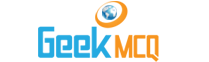# Entry Test MCQ :: Average

@ : Home > Math > Average

1.  Ahmad bought color pencils with price 3.45 16.75 9.84 and 8.16. Find the average amount spent on a pencil.
 A. 7.8 B. 8.2 C. 9.55 D. 18.2 E. none

2.  If X persons are admitted in a hospital during the last five years and Y persons are recovered out of them during this period then find the average number of persons admitted in one year:
 A. x/y B. (x-y)/5 C. x/5 D. (x+y)/5 E. none

3.  In the above question what is the average of un-recovered persons per year:
 A. (x-y)/5 B. x-y C. y/x D. (x+y)/5 E. none

4.  A family with six members has an average age of 25 while a family of 9 has an average age of 35. The combine average of both families will be:
 A. 30 B. 34.93 C. 39 D. 43 E. none

5.  The average of crimes per week in a city is A. Find the number of crimes in 4 weeks.
 A. A/4 B. 4A/7 C. 4A D. 7A/4 E. none

6.  A family of 6 has an average 25 and another of 9 has an average 41. The sum of the ages of the family members of both will be:
 A. 165 B. 375 C. 455 D. 615 E. none

7.  There are 45 persons staying at a hostel. The average expenses of a person for a day are rupees 20. The total expenses for all the persons for a week are:
 A. 295 B. 900 C. 9000 D. 6300 E. none

8.  The average earning of a shop for first three weeks of a month are 2750 per week. If the earnings of next 2 weeks are 4750 rupees then find total earnings of the 5 weeks.
 A. 3000 B. 17500 C. 17750 D. 13000 E. none

9.  The average height of a class of 18 students is 105 cm. After the arrival of 7 new students the average decreases by 5cm. Find sum of the heights of all the students in the class.
 A. 1890 B. 2500 C. 2625 D. 2890 E. none

10.  In the above question the sum of the height of new students in class.
 A. 190 B. 610 C. 710 D. 1890 E. none